# Division of Fractions

Go back to  'Fractions'

## Dividing a fraction by a whole number

What does \begin{align} \frac{1}{8} \div 2 \end{align} mean? It means, \$we are distributing/dividing \begin{align} \frac{1}{8} \end{align} litres of water among $$2$$ vessels.

Let us represent \begin{align} \frac{1}{8} \end{align} litres of water visually.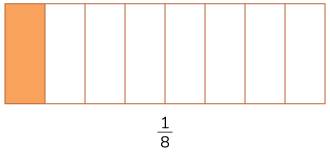Equally dividing the water we have, among two vessels means that each vessel gets,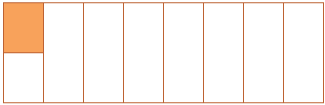But, if we are to tell what fraction of our water was poured into each vessel, we need to know how large the shaded portion is in this figure. And this becomes obvious only when the whole is divided into parts of the same size as our shaded portion.

Doing so, we get,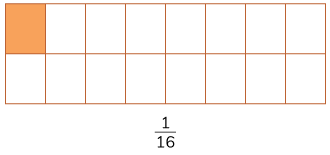One out of the $$16$$ parts is shaded – which means that each vessel gets \begin{align} \frac{1}{16} \end{align}  litres of water.

It can also be written as,

\begin{align} \frac{1}{8} \div 2 = \frac{1}{8} \times \frac{1}{2} = \frac{1}{16} \end{align}

This method is also known as ‘Reciprocal method’.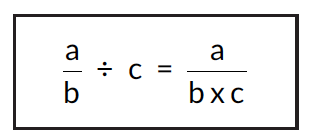## Dividing a whole number by a fraction

What does it mean to divide a whole number by a fraction? Let us consider dividing $$8$$ by \begin{align} \frac{1}{2}. \end{align}

It means distributing/dividing $$8$$ litres of water among vessels \begin{align} \frac{1}{2} \end{align} litres in size/volume. How many such \begin{align} \frac{1}{2} \end{align} litre vessels are needed? To answer this, let’s see how many \begin{align} \frac{1}{2}. \end{align} litre vessels are needed to fill a $$1$$ litre bucket. The answer is $$2.$$

There are $$16$$ one-half litres in $$8$$ litres. Hence we would need $$16$$ half litre vessels to equally distribute $$8L$$ of water.

By the reciprocal method it would be,

\begin{align} 8 \div \frac{1}{2} = \frac{8 \times 2}{1} = 16 \end{align}

It can be simply put as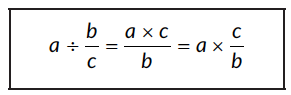## Dividing a fraction by another fraction

What does it mean to divide a fraction by a fraction? Let us consider \begin{align} \frac{1}{2} \div \frac{1}{4}. \end{align} It means dividing \begin{align} \frac{1}{2} \end{align} into pieces each \begin{align} \frac{1}{4} \end{align} units in size. Or alternately, we can consider this as a situation in which we are distributing/dividing \begin{align} \frac{1}{2} \end{align} litres of water among vessels \begin{align} \frac{1}{4} \end{align} litres in size/volume.

How many \begin{align} \frac{1}{4} \end{align} litre vessels are needed? The answer is $$2.$$

By the reciprocal method,

\begin{align} \frac{1}{2} \div \frac{1}{4} = \frac{1}{2} \times \frac{4}{1} = \frac{4}{2} = 2 \end{align}

The reciprocal method is simple and easy to use, simply put it is,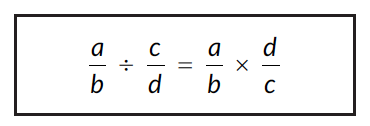## Tips and Tricks

• Tip: Always take the reciprocal first and switch the division sign with multiplication before simplifying. That way you dont have to consider whether you are simplifying one of the given fractions of across both of them.
• Remember, after taking the reciprocal you have a multiplication statement. Now simplification can also be done across the two fractions. E.g. if there is a common factor between the numerator of one of the fractions and the denominator of the other fraction, you can simplify them and proceed.
E.g. \begin{align} \frac{5}{28} \times \frac{7}{9} \end{align} can be simplified to \begin{align} \frac{5}{4} \times \frac{1}{9} \end{align} before multiplying.

### Common mistakes or misconceptions

• Children may simply replace the division symbol with a multiplication symbol and solve the problem. This happens when they try and “remember” the rule instead of understanding the concept. They know that the operation changes but do not understand why and end up blindly replacing the symbol and getting an incorrect answer.
• Children may “cancel” common factors across the two fractions before taking the reciprocal.Students are expected to take the reciprocal of the second fraction and switch the division sign with multiplication. While simplifying each fraction before this step won't change the answer, simplification across the two fractions (the numerator of one and the denominator of the other) will lead to an error.

## Divide

Q1. \begin{align} \frac{5}{6} \div 2 \end{align}
Q2. \begin{align} 5\frac{3}{5} \div 7 \end{align}
Q3. \begin{align} 2\frac{1}{3} \div 2 \end{align}